# Material Properties

View and edit thermal, rheological, mechanical, and PVT properties for materials.

## Materials Database

Use the Materials Database tab on the Materials dialog to view the material properties of any material in Inspire Mold. Click Edit to modify a material's properties and save a custom version of the material on the My Materials tab. You can also Import and Export materials to and from the database.

To view a material's properties, select the Material Group and Name at the top of the Materials dialog: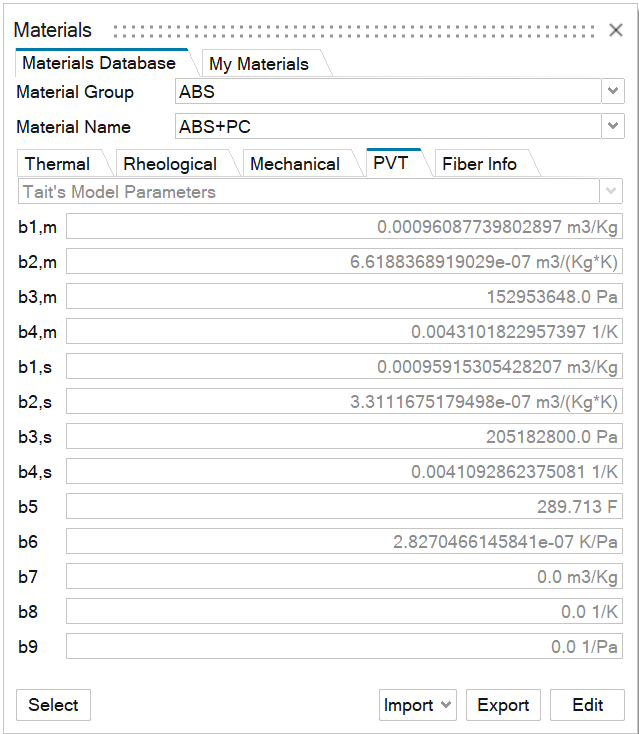Material Property Description
Material Group Lists the materials available in the Materials Database.
Material Name Lists the common polymers available for the specified material group.

## Description of Material Data

The injection molding solver requires the material properties of the polymer, mold, mold insert, part insert, and fiber to be specified.

The polymer material data consists of:
• Physical and thermal properties
• Equation of State - PVT data
• Rheological (constitutive) properties
• Mechanical properties
The mold, mold insert, and part insert material data consists of:
• Physical and thermal properties
• Mechanical properties

## Data Format and Units

• The current version of the solver expects the material data in .json format,
• All parameters are input in SI units

## Polymer Data

It is assumed that the polymer data is measured with its fillers and other additives.

## Physical and Thermal Properties

Each property can be specified as a scalar or as a table when considering variations due to temperature changes. Review the properties on the Thermal tab for the specified material:

Material Property Description
Specific Heat Specifies the heat energy required to increase the temperature of a unit quantity J/(Kg-K). Specific heat of the polymer can be specified as a scalar value or as a function of temperature in the form of a table.

Solver keyword: SPECIFIC_HEAT

Conductivity (Thermal Conductivity) Specifies the heat conductivity of the material (W/m-K). Thermal conductivity of the polymer can be specified as a scalar value or as a function of temperature in the form of a table.

Solver keyword: CONDUCTIVITY

Density Specifies the density of the polymer melt at the reference temperature in (kg/m3). Melt density is assumed to remain constant during the filling phase. During packing simulation, Tait 2-phase model for the equation of state is used to compute polymer density.

Solver keyword: DENSITY

## Rhealogical Properties

The solver models the rheology of the polymer using generalized Newtonian models. Models currently available in the solver compute viscosity as a function of effective shear rate and temperature. All the constitutive models are set by defining an appropriate combination of : a) an empirical model relating viscosity with shear rate and b) the temperature dependence. Temperature dependence is denoted using the symbol αT.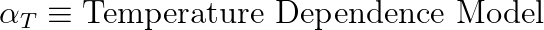Review the properties on the Rhealogical tab for the specified material:
Material Property Description
Constitutive Model Five models are included in the Materials Database. Select the model appropriate for your material choice. Review the Exponent, Consistency and Relative Shear Stress for your chosen model.
• Power Law Model
This model describes the shear rate dependency of viscosity using a power-law. The exponent n determines the nature of the relationship. This is a two-parameter model and it is described by the following equation: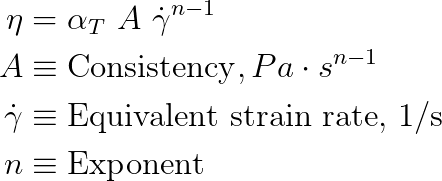The exponent affects the way the material viscosity changes with the shear rate in the following way:
• n < 1 Shear thinning or pseudoplastic – viscosity decreases as shear rate increases
• n > 1 Shear thickening or dilatant – viscosity increases as shear rate increases
• n = 1 Newtonian
Solver keywords: PowerLawModel, POWER_LAW_CONSISTENCY, POWER_LAW_EXPONENT
• Regularized Herschel-Bulkley Model
The Herschel-Bulkley model is used to represent the rheological behavior of polymers that have yield stress and exhibit shear thinning behavior when subjected to higher stresses. It is a four-parameter model and it is given by the following equation: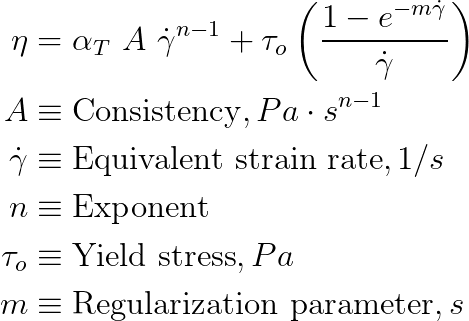• This model describes the viscosity using a power-law relationship and a yield criterion
• Yield criterion includes yield stress and a regularization parameter
• Regularization parameter controls the exponential growth of the stress at low shear rates
Database keywords: HerschelBulkleyModel, HB_CONSISTENCY, HB_EXPONENT, HB_YIELD_STRESS, HB_REGULARIZATION_PARAMETER
• Carreau-Yasuda
This is a well defined five-parameter model.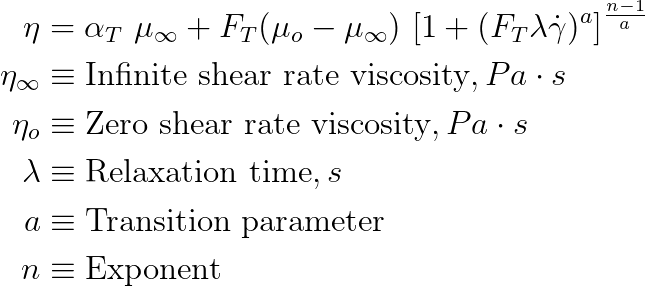As the shear rate becomes smaller, the viscosity function approaches a constant value: zero shear viscosity. Also, as the shear rate becomes larger, viscosity approaches another limit: infinite shear viscosity. This model also includes a time constant for the fluid to describe nonlinear shear rate dependence of viscosity. As the time constant approaches zero, the polymer behaves like a Newtonian fluid; higher values of time constant indicates a highly nonlinear relationship between viscosity and shear rate. Exponent n describes the slope of the power-law region.

Database keywords: CarreauYasudaModel, CY_INFINITE_SHEAR_VISCOSITY, CY_ZERO_SHEAR_VISCOSITY, CY_TIME_CONSTANT, CY_TRANSITION_PARAMETER, CY_EXPONENT

• Cross Model
The Cross model is widely used to describe the most common injection molded thermoplastics. It describes the viscosity-shear rate relationship using three parameters: consistency, reference shear stress, and exponent. The viscosity is computed using the following equation: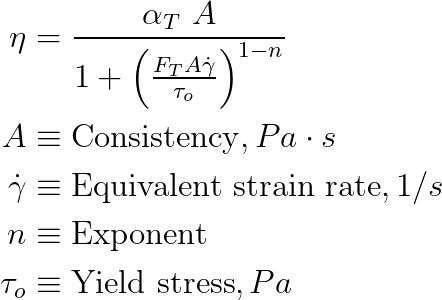• Consistency is the zero shear viscosity at the reference temperature
• Reference shear stress is the shear stress at the transition from the Newtonian region
• Exponent n is the power law index which accounts for shear thinning behavior

Database keywords: CrossModel, CROSS_CONSISTENCY, CROSS_EXPONENT, CROSS_REFERENCE_SHEARSTRESS

• Modified Cross Model
This is one of the most commonly used model. Parameters are similar to the cross model.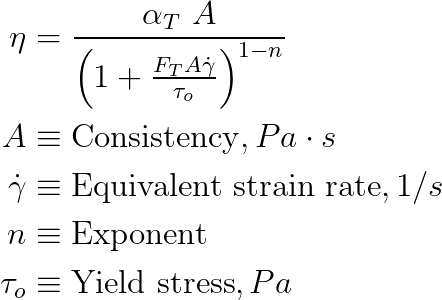Database keywords: ModifiedCrossModel, MODIFIED_CROSS_CONSISTENCY, MODIFIED_CROSS_EXPONENT, MODIFIED_ CROSS_REFERENCE_SHEARSTRESS

Find more information on Rheological Properties in the PDF: Materials Example and Reference.
Temperature Dependence Temperature dependency of the viscosity can be modeled through one of the following ways. The chosen model is specified with the temperature dependence parameter, which is set with an integer value from 0 to 3:
• None - No Dependence
• Viscosity is assumed to be independent of temperature. The output of the viscosity model is used directly, with no reducing/increasing factor.
• Temperature dependence = 0

Database keywords: TEMPERATURE_DEPENDENCE: 0

• Exponential Model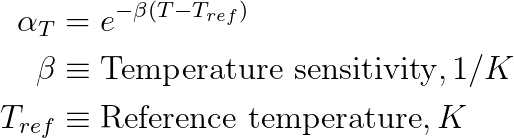• Temperature dependence = 1

Solver keywords: TEMPERATURE_DEPENDENCE: 1, BETA, REFERENCE_TEMPERATURE

• WLF (William-Landel-Ferry) Model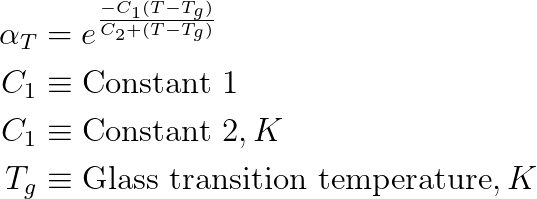• Glass transition temperature is computed by the following equation, taking pressure dependency into account: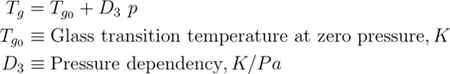• Temperature dependence = 2

Solver keywords: TEMPERATURE_DEPENDENCE: 2, WLF_CONSTANT_C1, WLF_CONSTANT_C2, WLF_GLASS_TRANSITION_TEMPERATURE, WLF_PRESSURE_DEPENDENCY_D3

• Arrhenius Model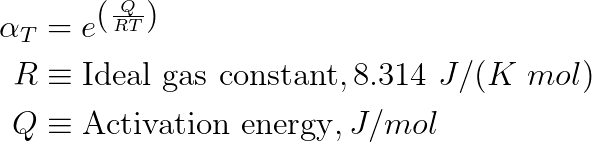• Temperature dependence = 3
• The only parameter that needs to be included in the material database is the activation energy
• This function is one of the commonly used methods to describe the variation of a property with respect to temperature

Database keywords: TEMPERATURE_DEPENDENCE: 3, ACTIVATION_ENERGY

Miscellaneous Review these parameters:
• Reference Temperature (K)
• No Flow Temperature: Temperature at which the material stops flowing. Generally, this is the Glass Transition temperature (Tg).
• Minimum Strain Rate Limit: Maximum shear rate for which the data is considered valid.
• Air Density: Assumed density of the air being displaced in the mold during filling (kg/m3).
Fiber Info Inlet fiber distribution is assumed to be random. Review the fiber content for the specified material including:
• Fiber Percentage: Percentage of fiber in the melt by weight.

Solver keyword: FIBER_PERCENTAGE

• Fiber Minimum Length : Minimum length of the fiber in meters.

Solver keyword: FIBER_MINIMUM_LENGTH

• Fiber Maximum Length Maximum length of the fiber in meters.

Solver keyword: FIBER_MAXIMUM_LENGTH

• Fiber Average Diameter:

Average diameter of the fiber in meters.

Solver keyword: FIBER_AVERAGE_DIAMETER
Note: Some materials do not include fiber orientation data. To add this data, edit an existing material and save it as a new material in the My Materials tab.

## Mechanical Properties

The solver uses Young's modulus, Poisson's ratio, and the thermal expansion coefficient to perform warpage calculations of the solidified part. Review the properties on the Mechanical tab for the specified material:

Material Property-Isotropic Model Description
Young's Modulus

Young's modulus of a material is a measure of the resistance of the material to deformation when subjected to stress. It is a measure of stiffness of the material.

Units: Pa

Solver keyword: YOUNG_MODULUS.
Poisson Ratio

Poisson's ratio is the ratio of lateral and longitudinal strain when subjected to uniaxial stress.

Solver keyword: POISSON_RATIO
Yield Stress

Specifies the stress point at which the material deformation changes from elastic to plastic.

Units: Pa

Coefficient of Thermal Expansion

The thermal expansion coefficient describes the extent of changes in the size of the object due to variation in temperature:

Units: 1/K

Solver keyword: COEFFICIENT_THERMAL_EXPANSION

## Equation of State - PVT Data

The pressure-volume-temperature (PVT) relationship is modeled using Tait's two-phase equation of state. The PVT behavior of the polymer in both solid and melt state is described using 14 parameters. These parameters are indicated with letter b and with a numerical subscript (1-9) and a letter subscript (m=melt, s=solid). The following equations describe the equation of state and its parameters.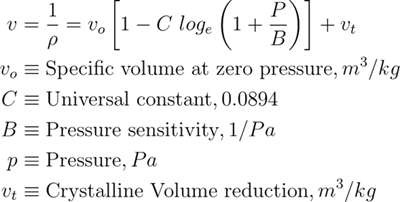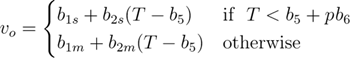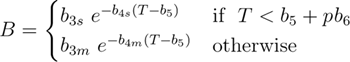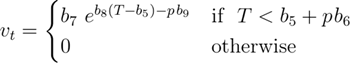Parameters b5 to b9 are identical for both melt and solid. They are repeated for simplicity of the data file and structure of the code.

TAIT_PARAMETERS_MOLTEN_STATE = [b1m , b2m, b3m, b4m, b5, b6, b7, b8, b9]

TAIT_PARAMETERS_SOLID_STATE = [b1s, b2s, b3s, b4s, b5, b6, b7, b8, b9]

Database keywords: TAIT_PARAMETERS_MOLTEN_STATE, TAIT_PARAMETERS_SOLID_STATE

## Processing Data

Melting Temperature:
• Valid for semi-crystalline materials
• The temperature at which the crystalline structures melt
• The solver does not use this. Will become useful when we implement crystallization kinetics
• Solver keyword: MELTING_TEMPERATURE
No Flow Temperature:
• The temperature at which material ceases to flow
• It is greater than Glass Transition Temperature
• Solver keyword: NO_FLOW_TEMPERATURE
Ejection Temperature:
• The temperature at which the part can be safely ejected
• Solver keyword: EJECTION_TEMPERATURE
Minimum Mold Temperature:
• Recommended minimum mold temperature
• Solver keyword: MINIMUM_MOLD_TEMPERATURE
Maximum Mold Temperature:
• Recommended maximum mold temperature
• Solver keyword: MAXIMUM_MOLD_TEMPERATURE
Minimum Melt Temperature:
• Recommended minimum melt temperature
• Solver keyword: MINIMUM_MELT_TEMPERATURE
Maximum Melt Temperature:
• Recommended maximum melt temperature
• Solver keyword: MAXIMUM_MELT_TEMPERATURE
Absolute Maximum Melt Temperature:
• The absolute maximum allowed for the melt temperature at the inlet
• Solver keyword: ABSOLUTE_MAXIMUM_MELT_TEMPERATURE

## Mold / Mold Insert / Part Insert Data

Reference Temperature:
• Reference Temperature at which the data is measured. Units: K
• Solver keyword: REFERENCE_TEMPERATURE

Density:
• Density of the material at reference temperature. Solver will also support a temperature dependent table in the place of a single value at the reference temperature. Units: kg/m3
• Solver keyword: DENSITY
Specific Heat:
• Specific heat of the material at reference temperature. Solver will also support a temperature dependent table in the place of a single value at the reference temperature. Units: J/(kg-K)
• Solver keyword: SPECIFIC_HEAT
Conductivity (Thermal Conductivity):
• Thermal conductivity of the material at reference temperature. Solver will also support a temperature dependent table in the place of a single value at the reference temperature. Units: W/(m-K)
• Solver keyword: CONDUCTIVITY
Young's Modulus:
• Young's modulus of the material. Units: Pa
• Solver keyword: YOUNG_MODULUS
Poisson Ratio:
• Poisson's ratio of the material.
• Solver keyword: POISSON_RATIO
Thermal expansion coefficient:
• The thermal expansion coefficient of the material. Units: 1/K
• Solver keyword: COEFFICIENT_THERMAL_EXPANSION

## Fiber Material data - For Stress Analysis of the Molded Part

This data is independent of the polymer or the percentage of weight in the mix. This is the basic fiber material data.

Reference Temperature:
• Reference Temperature at which the data is measured. Ideally it should be temperature at which the test is performed. Units: K
• Solver keyword: REFERENCE_TEMPERARTURE

Density:
• Density of the fiber at reference temperature. Solver will also support a temperature dependent table in the place of a single value at the reference temperature. Units: kg/m3
• Solver keyword: DENSITY
Specific Heat:
• Specific heat of the fiber at reference temperature. Solver will also support a temperature dependent table in the place of a single value at the reference temperature. Units: J/(kg-K)
• Solver keyword: SPECIFIC_HEAT
Conductivity (Thermal Conductivity):
• Thermal conductivity of the fiber at reference temperature. Solver will also support a temperature dependent table in the place of a single value at the reference temperature. Units: W/(m-K)
• Solver keyword: CONDUCTIVITY
Young's Modulus:
• Young's modulus of the fiber. Units: Pa
• Solver keyword: YOUNG_MODULUS
Poisson Ratio:
• Poisson's ratio of the fiber.
• Solver keyword: POISSON_RATIO
Thermal expansion coefficient:
• The thermal expansion coefficient of the fiber. Units: 1/K
• Solver keyword: COEFFICIENT_THERMAL_EXPANSION

## Base Polymer Data For Stress Analysis of the Molded Part

Reference Temperature:
• Reference Temperature at which the data is measured. Units: K
• Solver keyword: REFERENCE_TEMPERATURE
Density:
• Density of the material at reference temperature. Solver will also support a temperature dependent table in the place of a single value at the reference temperature. Units: kg/m3
• Solver keyword: DENSITY
Specific Heat:
• Specific heat of the material at reference temperature. Solver will also support a temperature dependent table in the place of a single value at the reference temperature. Units: J/(kg-K)
• Solver keyword: SPECIFIC_HEAT
Conductivity (Thermal Conductivity):
• Thermal conductivity of the material at reference temperature. Solver will also support a temperature dependent table in the place of a single value at the reference temperature. Units: W/(m-K)
• Solver keyword: CONDUCTIVITY
Young's Modulus:
• Young's modulus of the material. Units: Pa
• Solver keyword: YOUNG_MODULUS
Poisson Ratio:
• Poisson's ratio of the material.
• Solver keyword: POISSON_RATIO
Thermal expansion coefficient:
• The thermal expansion coefficient of the material. Units: 1/K
• Solver keyword: COEFFICIENT_THERMAL_EXPANSION

## Unit Summary

Data Unit
Density kg/m3
Specific Heat J/(kg-K)
Conductivity W/(m-K)
Yield Stress Pa
Young Modulus Pa
Poisson Ratio -
Coefficient Thermal Expansion 1/K
Reference Temperature K
Minimum Mold Temperature K
Maximum Mold Temperature K
Minimum Melt Temperature K
Maximum Melt Temperature K
Glass Transition Temperature K
No Flow Temperature K
Ejection Temperature K
Viscosity model:
Modified Cross: -
Consistency Pa.s
Exponent -
Reference Shear stress (tau) Pa
Zero Shear Viscosity Pa.s
Infinite Shear Viscosity Pa.s
Min Strainrate Limit 1/s
Max Strainrate Limit 1/s
Temperature Dependence:
WLF:
Constant C1 -
Constant C2 K
Pressure Dependency D3 K/Pa
Tait Parameters:
• b1m, b1s
• b2m, b2s
• b3m, b3s
• b4m, b4s
• b5
• b6
• b7
• b8
• b9

• m3/kg
• m3/kg K
• Pa
• 1/K
• K
• K/Pa
• m3/kg
• 1/K
• 1/Pa
Filler Data:
Fiber Percentage Percentage of fiber in the melt by weight
Fiber Minimum Length m
Fiber Maximum Length m
Fiber Average Diameter m